Plotting in Julia¶

There are an intimidating amount of plotting packages¶

Luckily, we have Plots.jl.

Plots.jl is a plotting metapackage which brings many different plotting packages under a single API, making it easy to swap between plotting "backends".

They all have their pros and cons. You can explore some here:

https://docs.juliaplots.org/latest/backends¶Today, we will focus on PyPlot.

Pros:

• Tons of functionality
• 2D and 3D
• Mature library
• Well supported in Plots.jl

Cons:

• Uses python
• Dependencies frequently cause setup issues
• Inconsistent output depending on Matplotlib version

Installation¶

Unfortunately, you need not only Python but some of the SciPy ecosystem. You can simply install everything needed by installing Anaconda (https://conda.io/docs/user-guide/install/index.html).

To install the Plots package, in Julia simply type

In [ ]:
using Pkg

And of course you will need some backend, so type

In [ ]:

In :
## Now we are ready to get started!
using Plots
In :
## Lets jump right in
data = rand(10)
f = plot(data)
@show f
f = Plot{Plots.GRBackend() n=1}
Out:

We didn't specify a backend! Mine defaulted to GR. Let's tell it to use pyplot.

In :
Plots.pyplot()
f = plot(data);
@show f
f = Plot{Plots.PyPlotBackend() n=1}
Out: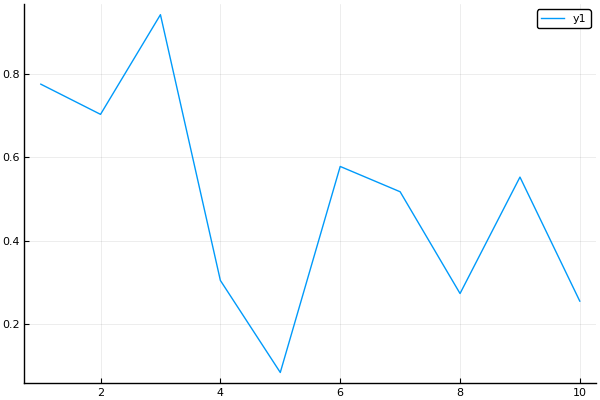You can add more than 1 graph to the same plot:

In :
# In Plots.jl, every column is a 'series'
data2 = rand(10, 2); # 10x2 random mat
plot(data2)

# not necessary to use a variable f = ...
Out: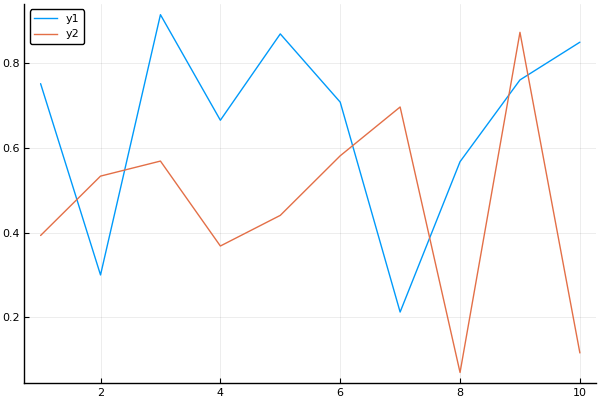In :
# You can also add to the most recent plot using plot!()
plot!(rand(10))
Out: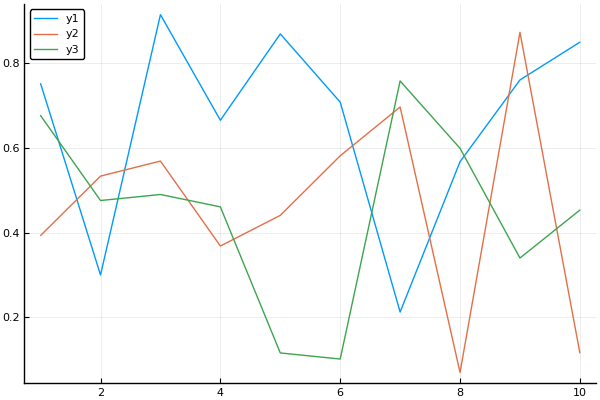In :
# or add to a specific plot
plot!(f,rand(10))
Out: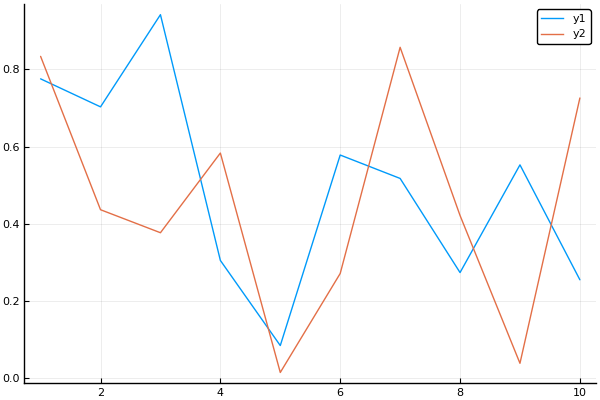Attributes¶

aka how to make your graph pretty¶

Julia calls things like color, line width, etc. 'Attributes'.

In general, these are set by keyword arguements.

i.e. plot(data, keyword=value)

For a long list of Attributes, check out: http://docs.juliaplots.org/latest/attributes/

And check http://docs.juliaplots.org/latest/supported/ to see if your backend supports those Attributes

Lets Play around with some Attributes¶

First: linestyle, color, and markershape

In :
# tip: use Plots.supported_styles() or Plots.supported_markers() to see which linestyles or markershapes you can use
@show Plots.supported_styles();
@show Plots.supported_markers();
Plots.supported_styles() = Symbol[:auto, :solid, :dash, :dot, :dashdot]
Plots.supported_markers() = Symbol[:none, :auto, :circle, :rect, :star5, :diamond, :hexagon, :cross, :xcross, :utriangle, :dtriangle, :rtriangle, :ltriangle, :pentagon, :heptagon, :octagon, :star4, :star6, :star7, :star8, :vline, :hline, :+, :x, :pixel]
In :
plot(data2, markershape = :ltriangle, linestyle = :dashdot, color = [:black :orange])
Out: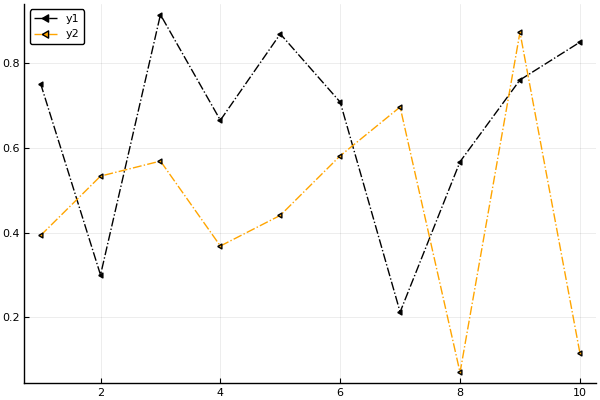'c' argument looks like a single numeric RGB or RGBA sequence, which should be avoided as value-mapping will have precedence in case its length matches with 'x' & 'y'.  Please use a 2-D array with a single row if you really want to specify the same RGB or RGBA value for all points.
'c' argument looks like a single numeric RGB or RGBA sequence, which should be avoided as value-mapping will have precedence in case its length matches with 'x' & 'y'.  Please use a 2-D array with a single row if you really want to specify the same RGB or RGBA value for all points.

This is a prime example of matplotlib dependencies raising errors

In :
# You can use ! to update the last plot with certain Attributes

plot!(title="Test Plot")
Out: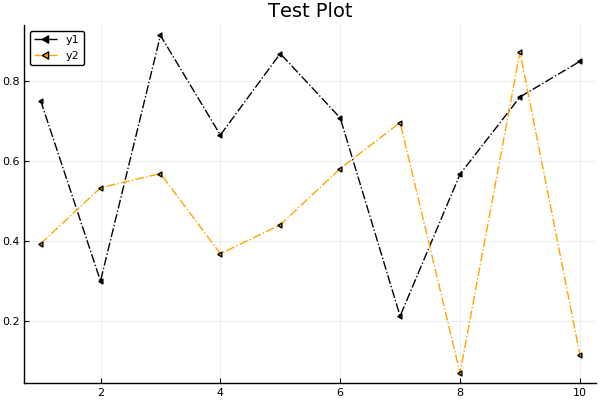'c' argument looks like a single numeric RGB or RGBA sequence, which should be avoided as value-mapping will have precedence in case its length matches with 'x' & 'y'.  Please use a 2-D array with a single row if you really want to specify the same RGB or RGBA value for all points.
'c' argument looks like a single numeric RGB or RGBA sequence, which should be avoided as value-mapping will have precedence in case its length matches with 'x' & 'y'.  Please use a 2-D array with a single row if you really want to specify the same RGB or RGBA value for all points.
In :
# Certain attributes have their own modifier function (!) too

plot!(ylabel = "y axis")
xlabel!("x axis")

#same result
Out: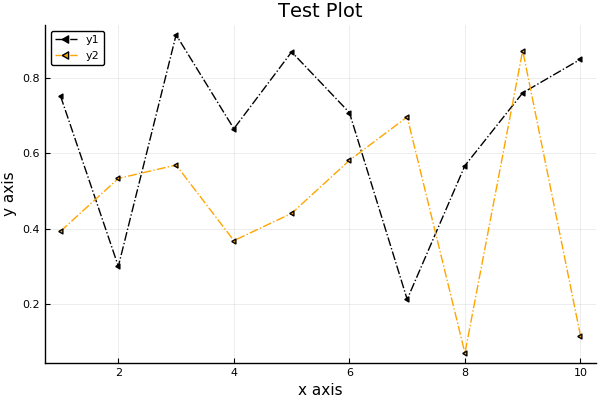'c' argument looks like a single numeric RGB or RGBA sequence, which should be avoided as value-mapping will have precedence in case its length matches with 'x' & 'y'.  Please use a 2-D array with a single row if you really want to specify the same RGB or RGBA value for all points.
'c' argument looks like a single numeric RGB or RGBA sequence, which should be avoided as value-mapping will have precedence in case its length matches with 'x' & 'y'.  Please use a 2-D array with a single row if you really want to specify the same RGB or RGBA value for all points.

There are also these 'magic' attributes (e.g. axis, xaxis, marker, line) where you can pass multiple sub-attributes at once.

You don't even have to label your sub-attributes. Julia does some type-checking magic to figure out which value applies to which argument.

Lets test this out¶
In :
# lets use new data

data3 = hcat(Array(0:0.01:1),Array(1:-0.01:0)) #concatenate along dimension 2
data3 += .05*randn(size(data3)) # lets add randomness so its not so boring
Out:
101×2 Array{Float64,2}:
0.0265667   0.991724
0.0933707   1.07117
0.0415541   1.0346
0.101022    1.05393
0.124824    0.959763
0.0710637   0.889158
0.086548    0.920414
0.0215248   0.989714
0.0374153   0.95194
0.0678798   0.906038
0.131726    0.938583
0.0702449   0.885036
0.170403    0.904721
⋮
0.844436    0.124202
0.977635    0.0991429
0.868893    0.0453534
0.917798    0.102463
0.876271    0.0552505
0.831023   -0.0216485
0.961214    0.021448
0.990352    0.110152
0.97341     0.0798246
0.915008   -0.0314549
1.02283     0.0537127
0.972636    0.0846006
In :
plot(data3, title="Pizza Intake vs Regret", xaxis = (font(5), "Slices Eaten", 0:25:101, :log10),
ylabel="Regret",line=(0.5, 3), label=["Charlie" "Omar"])

## Each attribute in xaxis = (font(32), "Slices Eaten", 0:25:101, :log10) has its own name.
## We could've assigned their values seperately, e.g.
## xtickfont = font(32), xlabel = "Slices Eaten", ...

## same with line=(0.5, 3)
Out: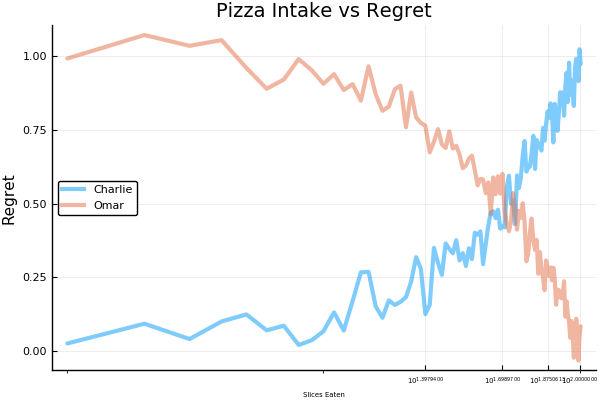To make all of these changes by hand (clicking your mouse), use gui()¶

doesn't work on Jupyter

In :
gui()
C:\Users\Tony\.julia\conda\3\lib\site-packages\matplotlib\figure.py:448: UserWarning: Matplotlib is currently using agg, which is a non-GUI backend, so cannot show the figure.
% get_backend())

Input arguments take many forms¶

In [ ]:
plot()                                    # empty Plot object
plot(4)                                   # initialize with 4 empty series
plot(rand(10))                            # 1 series... x = 1:10    <--------------------- we've used this
plot(rand(10,5))                          # 5 series... x = 1:10    <--------------------- and this
plot(rand(10), rand(10))                  # 1 series
plot(rand(10,5), rand(10))                # 5 series... y is the same for all
plot(sin, rand(10))                       # y = sin(x)
plot(rand(10), sin)                       # same... y = sin(x)
plot([sin,cos], 0:0.1:π)                  # 2 series, sin(x) and cos(x)
plot([sin,cos], 0, π)                     # sin and cos on the range [0, π]
In :
# you can pass in generic functions like:
sin
Out:
sin (generic function with 12 methods)
Does order of inputs matter?¶

Well, sometimes

In :
p1 = plot(sin, 0:0.01:2*pi)
p2 = plot(0:0.01:2*pi, sin)

x = Array(0:0.01:2*pi);
y = sin.(x); # elementwise sin(x)

p3 = plot(x,y)
p4 = plot(y,x)

plot(p1,p2,p3,p4)

# layout is:
# p1 p2
# p3 p4
Out: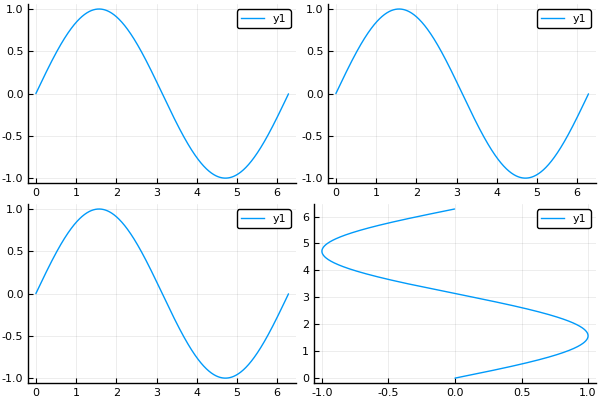Layout¶

The above plot defaulted to a 2x2 grid.

We can change this using the argument: layout = (4,1)

You can also add attributes as you did previously

In :
using LaTeXStrings

plot(p1,p2,p3,p4, layout = (4,1),
legend=[false true false true],
label = ["" "sin(x)" "" "arcsin(x)"], # using "" skips over that plot.
annotate = [(3,0,"sin(x)") (0,0,"") (3,0,text(L"sin(x)")) (0,0,"")]) # L"" uses LaTeX to construct string
Out: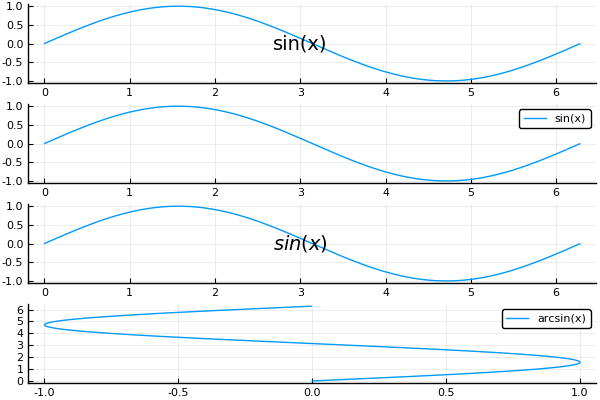In :
#Note: changing the attributes like this changes the original plots!
p1
Out: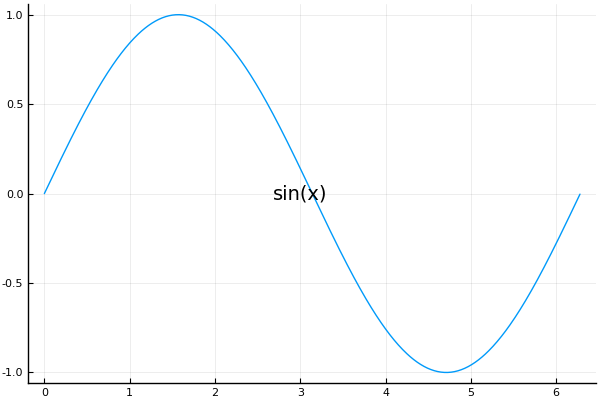Images¶

(a reason to show off my dogs)

In :
using Images #may need to Pkg.add("Images")